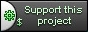# Starting with DbiXX

DbiXX is not a mandatory part of the CppCMS framework; it is just a general purpose library to execute SQL queries in a safe way.

Let's see a simple example. You can find the code of this example within the cppcms source code, at `cppcms/examples/dbixx/dbi.cpp` of CppCMS 1.x.x or in the independent set of examples downloadable from sourceforge.

```#include <dbixx/dbixx.h>
#include <iostream>

using namespace dbixx;
using namespace std;

int main()
{
try {
session sql("sqlite3");
sql.param("dbname","test.db");
sql.param("sqlite3_dbdir","./");
sql.connect();

sql<<"DROP TABLE IF EXISTS users";
sql.exec();
sql<<"CREATE TABLE users ( "
" id integer primary key not null, "
" name varchar(128) not null "
");";
sql.exec();
sql<<"INSERT INTO users(id,name) VALUES(?,?)",
1,"Moshe",exec();
sql<<"INSERT INTO users(id,name) VALUES(?,?)",
2,"Yossi",exec();
sql<<"SELECT name FROM users WHERE id=?",1;
row r;
if(sql.single(r)) {
string name;
r>>name;
cout<<name<<endl;
}
else {
cout<<"No user with id="<<1<<endl;
}
result res;
sql<<"SELECT id,name FROM users";
sql.fetch(res);
cout<<"There are "<<res.rows()<<" users\n";
while(res.next(r)) {
int id;
string name;
r>>id>>name;
cout<<id<<"\t"<<name<<endl;
}
}
catch(std::exception const &e) {
cerr<<e.what()<<endl;
return 1;
}
return 0;
}
```

First we create a sql session object and load the driver "sqlite3". Then we setup all the mandatory parameters needed by the driver and create a connection.

```session sql("sqlite3");
sql.param("dbname","test.db");
sql.param("sqlite3_dbdir","./");
sql.connect();
```

Then we perform queries. First we prepare them using "iostreams like" style:

```sql<<"DROP TABLE IF EXISTS users";
```

and then execute the operation:

```sql.exec();
```

There is a syntactic sugar for this operation:

```sql<<"DROP TABLE IF EXISTS users",exec();
```

Then we want to execute some commands using parameters binding:

```sql<<"INSERT INTO users(id,name) VALUES(?,?)",
1,"Moshe",exec();
```

First we load our query. Each "?" represents a bound parameter. Then, using overloaded comma operator, we bind actual values: the integer "1" and the string "Moshe". Note: every bound string is automatically escaped.

Now we want to fetch a single row of data. First, we bind the query and its parameters as before.

```sql<<"SELECT name FROM users WHERE id=?",1;
```

But now, we store the output data in a single row class.

```row r;
if(sql.single(r)) {
```

If the result wasn't an empty set, the condition is true and we can readout the data from the row, using the "iostreams" like interface.

```r>>name;
```

Now, we want to fetch some bigger data set. In this case we use the class result that stores the output data. We use:

```result res;
sql.fetch(res);
```

Now we can find out the number of rows calling `res.rows()` and iterate over each row calling `res.next(r)`.

Now, you can read the full DbiXX Library API

CppCMS is a web development framework for performance demanding applications.

## Support This Project